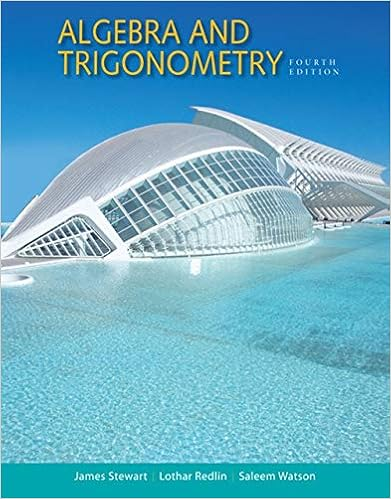# 3 2 1 2 x y x 2 4 y 2 20 1 x y 2 2 2 5 5 5 10 2 5 6 2

• Notes
• 56

This preview shows page 37 - 40 out of 56 pages.

##### We have textbook solutions for you!
The document you are viewing contains questions related to this textbook.The document you are viewing contains questions related to this textbook.
Chapter 5 / Exercise 14
Algebra and Trigonometry
Redlin/StewartExpert Verified
3 2 1 2 x y x' 2 —– 4 y' 2 —– 20 1 x' y' 2 2 2 5 5 5 ⎯⎯ 10 2 5 6 2 x 2 ⎯3 xy y 2 10 0 ⎯⎯ 10 Figure A5.26 This triangle identifies 2 a cot 1 1 3 as p 3. (Example 2) Figure A5.27 The conic section in Example 2.
##### We have textbook solutions for you!
The document you are viewing contains questions related to this textbook.The document you are viewing contains questions related to this textbook.
Chapter 5 / Exercise 14
Algebra and Trigonometry
Redlin/StewartExpert Verified
Section A5 Conic Sections 599 Now, the graph of Equation 11 is a (real or degenerate) (a) parabola if A or C 0; that is, if A C 0; (b) ellipse if A and C have the same sign; that is, if A C 0; (c) hyperbola if A and C have opposite signs; that is, if A C 0. It can also be verified from Equations 6 that for any rotation of axes, B 2 4 AC B 2 4 A C . (12) This means that the quantity B 2 4 AC is not changed by a rotation. But when we rotate through the angle a given by Equation 10, B becomes zero, so B 2 4 AC 4 A C . Since the curve is a parabola if A C 0, an ellipse if A C 0, and a hyperbola if A C 0, the curve must be a parabola if B 2 4 AC 0, an ellipse if B 2 4 AC 0, and a hyperbola if B 2 4 AC 0. The number B 2 4 AC is called the discriminant of Equation 9. EXAMPLE 3 Applying the Discriminant Test (a) 3 x 2 6 xy 3 y 2 2 x 7 0 represents a parabola because B 2 4 AC 6 2 4 3 3 36 36 0. (b) x 2 xy y 2 1 0 represents an ellipse because B 2 4 AC 1 2 4 1 1 3 0. (c) xy y 2 5 y 1 0 represents a hyperbola because B 2 4 AC 1 2 4 0 1 1 0. Now try Exercise 15. Technology Application How Some Calculators Use Rotations to Evaluate Sines and Cosines Some calculators use rotations to calculate sines and cosines of arbitrary angles. The pro- cedure goes something like this: The calculator has, stored, 1. ten angles or so, say a 1 sin 1 10 1 , a 2 sin 1 10 2 , … , a 10 sin 1 10 10 , and 2. twenty numbers, the sines and cosines of the angles a 1 , a 2 , … , a 10 . To calculate the sine and cosine of an arbitrary angle u , we enter u (in radians) into the calculator. The calculator substracts or adds multiples of 2 p to u to replace u by the angle between 0 and 2 p that has the same sine and cosine as u (we continue to call the angle u ). Discriminant Test With the understanding that occasional degenerate cases may arise, the quadratic curve Ax 2 Bxy Cy 2 Dx Ey F 0 is (a) a parabola if B 2 4 AC 0, (b) an ellipse if B 2 4 AC 0, (c) a hyperbola if B 2 4 AC 0.
600 Appendices Table A5.3 Examples of quadratic curves Ax 2 Bxy Cy 2 Dx Ey F 0 A B C D E F Equation Remarks Circle 1 1 4 x 2 y 2 4 A C ; F 0 Parabola 1 9 y 2 9 x Quadratic in y , linear in x Ellipse 4 9 36 4 x 2 9 y 2 36 A , C have same sign, A C ; F 0 Hyperbola 1 1 1 x 2 y 2 1 A , C have opposite signs One line (still 1 x 2 0 y -axis a conic section) Intersecting lines Factors to (still a conic 1 1 1 1 xy x y 1 0 x 1 y 1 0, section) so x 1, y 1 Parallel lines Factors to (not a conic 1 3 2 x 2 3 x 2 0 x 1 x 2 0, section) so x 1, x 2 Point 1 1 x 2 y 2 0 The origin No graph 1 1 x 2 1 No graph The calculator then “writes” u as a sum of multiples of a 1 (as many as possible without overshooting) plus multiples of a 2 (again, as many as possible), and so on, working its way to a 10 . This gives u m 1 a 1 m 2 a 2 m 10 a 10 .
•••